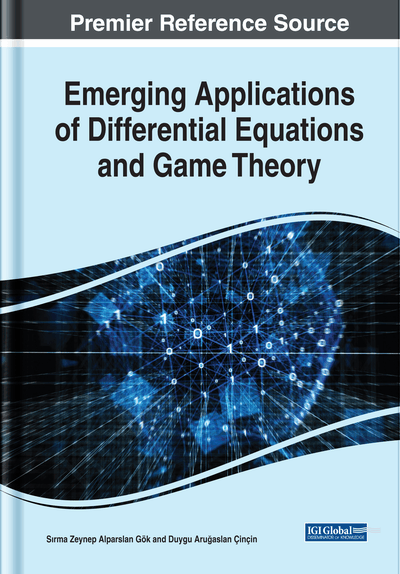# Spectral Problem for a Polynomial Pencil of the Sturm-Liouville Equations: On the Completeness of the System of Eigenfunctions and Associated Eigenfunction, Asymptotic Formula

Anar Adiloğlu-Nabiev (Süleyman Demirel University, Turkey)
DOI: 10.4018/978-1-7998-0134-4.ch008
Available
\$37.50
No Current Special Offers

## Abstract

A boundary value problem for the second order differential equation -y′′+∑_{m=0}N−1λ^{m}q_{m}(x)y=λ2Ny with two boundary conditions a_{i1}y(0)+a_{i2}y′(0)+a_{i3}y(π)+a_{i4}y′(π)=0, i=1,2 is considered. Here n>1, λ is a complex parameter, q0(x),q1(x),...,q_{n-1}(x) are summable complex-valued functions, a_{ik} (i=1,2; k=1,2,3,4) are arbitrary complex numbers. It is proved that the system of eigenfunctions and associated eigenfunctions is complete in the space and using elementary asymptotical metods asymptotic formulas for the eigenvalues are obtained.
Chapter Preview
Top

## Introduction

Consider the boundary value problem generating in the interval by the Sturm-Liouville pencil

(1) and two boundary conditions(2) where n>1, λ is a complex parameter, q0(x), q1(x),…,qn-1(x) are summable complex-valued functions, aik (i=1,2: k=1,2,3,4) are arbitrary complex numbers. In the case n>1 the equation (1) is a classical Sturm-Liouville equation and the spectral problem under boundary conditions (2) was completely studied in (Marchenko, 1997) where special integral representations called transformation operators for linearly independent solutions of the Sturm-Liouville equation are applied to investigate the spectral problem. In this monograph, using some important properties of the transformation operators, especially the relations between the potential of the Sturm-Liouville equation and the kernel of the transformation operators, the boundary value problem generated in a finite interval by the Sturm-Liouville equation and by regular boundary conditions of type (2) was investigated, the completeness of the system of eigenfunctions and associated eigenfunctions was proved and asymptotical formulas as λ→∞ was obtained for the solutions of the Sturm-Liouville equation. These asymptotic formulas of solutions are important to obtain the asymptotical formulas for the eigenvalues. The transformation operators allow to estimate the remainder part of the asymptotic formulas for the eigenvalues in relation with smoothness of the potential.

## Complete Chapter List

Search this Book:
Reset